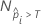# Methods and formulas for the gain chart for Fit Model and Discover Key Predictors with TreeNet® Classification

###### Note

The procedure for the points on the gain chart depends on the validation method. For a multinomial response variable, Minitab displays multiple charts that treat each class as the event in turn.

## No validation

For the chart for a training data set, each point on the chart represents a distinct fitted event probability. The highest event probability is the first point on the chart and appears leftmost. The other points on the chart are in order of decreasing event probability.

The y-coordinate of the gain chart is the true positive rate in a percent scale. The calculation of the true positive rate is the same as for the ROC curve chart. The x-coordinate of the chart has the following form:

whereis the number of rows where the fitted probability is greater than the threshold and N is the total number of rows. For details on the thresholds, go to Methods and Formulas for the Receiver Operating Characteristic (ROC) curve chart for Fit Model and Discover Key Predictors with TreeNet® Classification.

## Separate test set

Use the same steps as the training set case, but calculate the event probabilities from the cases for the test set.

## Test with k-fold cross-validation

Use the same steps as the training data set procedure, but calculate the event probabilities from the cases for the cross-validated data.